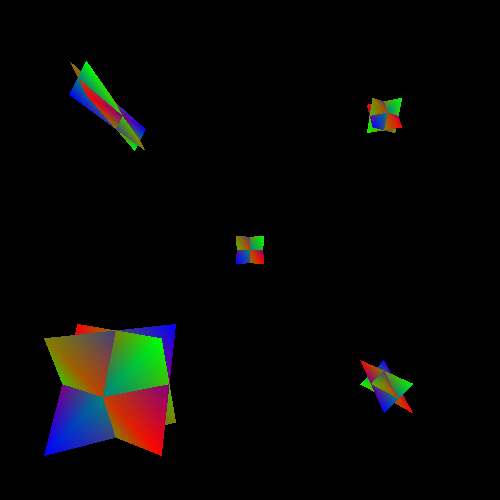## Scale

Another kind of transformation is scaling. In terms of our previous definition of a coordinate system, this means that our basis vectors are getting shorter or longer.

Figure 6.4. Coordinate System Scaling in 2DScaling can be uniform, which means each basis vector is scaled by the same value. A non-uniform scale means that each basis can get a different scale or none at all.

Uniform scales are used to allow objects in model space to have different units from the units used in camera space. For example, a modeller may have generated the model in inches, but the world uses centimeters. This will require applying a uniform scale to all of these models to compensate for this. This scale should be 2.54, which is the conversion factor from inches to centimeters.

Note that scaling always happens relative to the origin of the space being scaled.

Recall how we defined the way coordinate systems generate a position, based on the basis vectors and origin point:If you are increasing or decreasing the length of the basis vectors, this is the same as multiplying those basis vectors by the new length. So we can re-express this equation as follows:Since scalar-vector multiplication is both associative and commutative, we can multiply the scales directly into the coordinate values to achieve the same effect. So a scaled space can be reexpressed as simply multiplying the input coordinate values.

This is easy enough to do in GLSL, if you pass a vector uniform containing the scale values. But that's just not complicated enough. Obviously, we need to get matrices involved, but how?

This gets a bit technical, in terms of how a matrix multiplication works. But look back at the identity matrix:This matrix selects each coordinate in turn from the vector it is being multiplied into. Each row is multiplied with the column of the vector; all of the zeros remove the components of the vector that we do not want. The 1 value of each row multiplies into the component we do want, thus selecting it. This produces the identity result: the vector we started with.

We can see that, if the ones were some other value, we would get a scaled version of the original vector, depending on which ones were changed. Thus, a scaling transformation matrix looks like this:

Equation 6.4. Scaling Transformation MatrixYou may start to see a pattern emerging here, something that begins to suggest why matrices are very, very useful. I will not spoil it for you yet though.

The tutorial project Scale will display 5 objects at various scales. The objects are all at the same Z distance from the camera, so the only size difference between the objects is the scale effects applied to them. The object in the center is unscaled; each of the other objects has a scale function of some kind applied to them.

Figure 6.5. Scale ProjectOther than the way the tutorial builds its matrices, there is no difference between this tutorial project and the previous one. The matrix building code works as follows:

```glm::mat4 ConstructMatrix(float fElapsedTime)
{
glm::vec3 theScale = CalcScale(fElapsedTime);
glm::mat4 theMat(1.0f);
theMat.x = theScale.x;
theMat.y = theScale.y;
theMat.z = theScale.z;
theMat = glm::vec4(offset, 1.0f);

return theMat;
}```

As before, the scale is supplied by a number of scale functions, depending on which instance is being rendered. The scale is stored in the columns of the identity matrix. Then the translation portion of the matrix is filled in.

The `offset` variable is also a member of the `Instance` object. Unlike the last tutorial, the offset is a fixed value. We will discuss the ramifications of applying multiple transforms later; suffice it to say, this currently works.

Scaling is only slightly more complicated than translation.

### Inversion and Winding Order

Scales can be theoretically negative, or even 0. A scale of 0 causes the basis vector in that direction to become 0 entirely. An basis vector with no length means that a dimension has effectively been lost. The resulting transform squashes everything in that direction down to the origin. A 3D space becomes a 2D space (or 1D or 0D, depending on how many axes were scaled).

A negative scale changes the direction of an axis. This causes vertices transformed with this scale to flip across the origin in that axis's direction. This is called an inversion. This can have certain unintended consequences. In particular, it can change the winding order of vertices.

Back in Tutorial 4, we introduced the ability to cull triangles based on the order in which the vertices appeared in window space. Depending on which axis you negate, relative to camera space, an inversion can flip the expected winding order of vertices. Thus, triangles that were, in model space, forward-facing now in camera space are backwards-facing. And vice-versa.

Negative scaling can have other problems as well. This is not to say that inversions cannot be used, but they should be used with care.